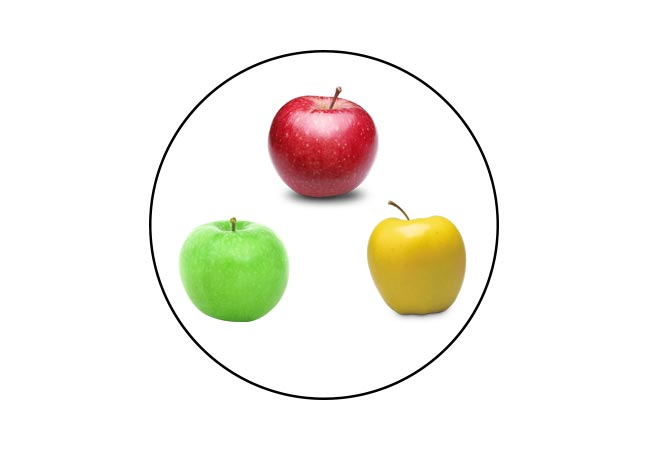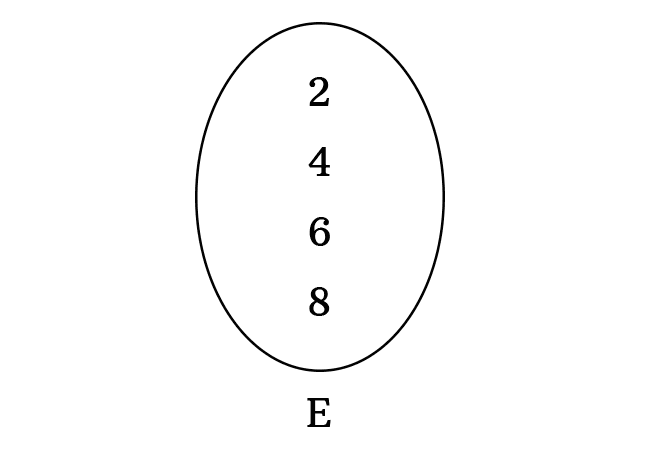# Elements of a Set

An object that forms a set is called an element (or a member) of a set.

## Introduction

The objects are collected on the basis of one or more common factors for defining a set in mathematics and every object is called an element of the set. It is also called as a member of a set. Now, let’s learn the concept of members of a set from three understandable examples.

### Basic exampleIn general, we buy or sell or carry the objects in closed packets. For example, three apples are collected in a closed geometric shape for a purpose. The collection of them formed a set. In this set, each object is called an element or a member.

1. The red apple is an element of the set.
2. The green apple is also a member of the set.
3. The yellow apple is also an element of the set.

### Numerical exampleMathematics is all about numbers. So, let’s understand the concept of elements or members of a set from a numerical example.

The objects $2$, $4$, $6$ and $8$ are basically numbers and they are collected to form a set. Actually, the four objects are even numbers and the set is denoted by a letter $E$.

In the set $E$, the objects $2$, $4$, $6$ and $8$ are called the elements of a set. They are also called as the members of the set $E$.In advanced mathematics, we use letters instead of numbers. Hence, the objects are denoted by the small (or lowercase) letters in set theory.

The objects $a$, $b$, $c$, $d$ and $e$ are five alphabets. The collection of them is formed a set $A$.

Now, the lowercase letters $a$, $b$, $c$, $d$ and $e$ are called the elements of set $A$. Similarly, they are also called as the members of the set $A$.

A best free mathematics education website that helps students, teachers and researchers.

###### Maths Topics

Learn each topic of the mathematics easily with understandable proofs and visual animation graphics.

###### Maths Problems

A math help place with list of solved problems with answers and worksheets on every concept for your practice.

Learn solutions

###### Subscribe us

You can get the latest updates from us by following to our official page of Math Doubts in one of your favourite social media sites.